# Types of Chemical Reactions and Solution Stoichiometry Aqueous

• Slides: 34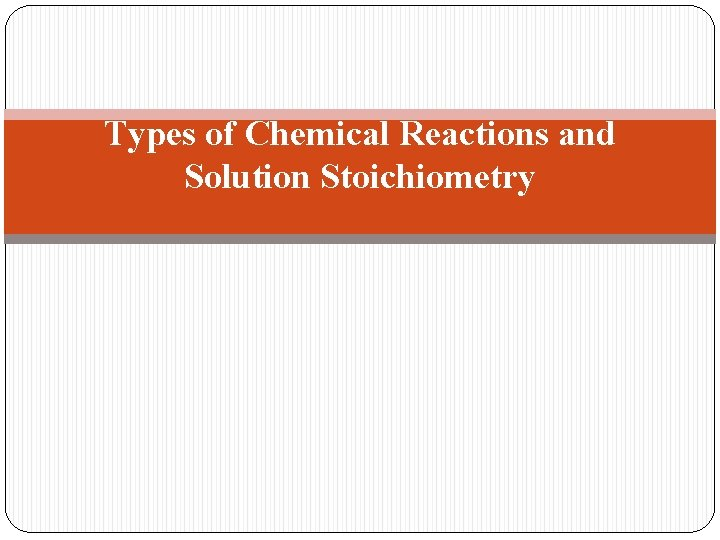Types of Chemical Reactions and Solution StoichiometryAqueous Solutions : Water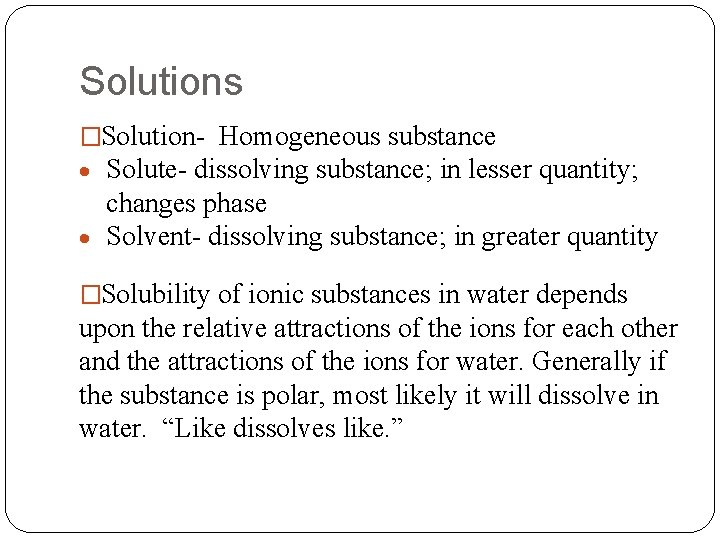Solutions �Solution- Homogeneous substance Solute- dissolving substance; in lesser quantity; changes phase Solvent- dissolving substance; in greater quantity �Solubility of ionic substances in water depends upon the relative attractions of the ions for each other and the attractions of the ions for water. Generally if the substance is polar, most likely it will dissolve in water. “Like dissolves like. ”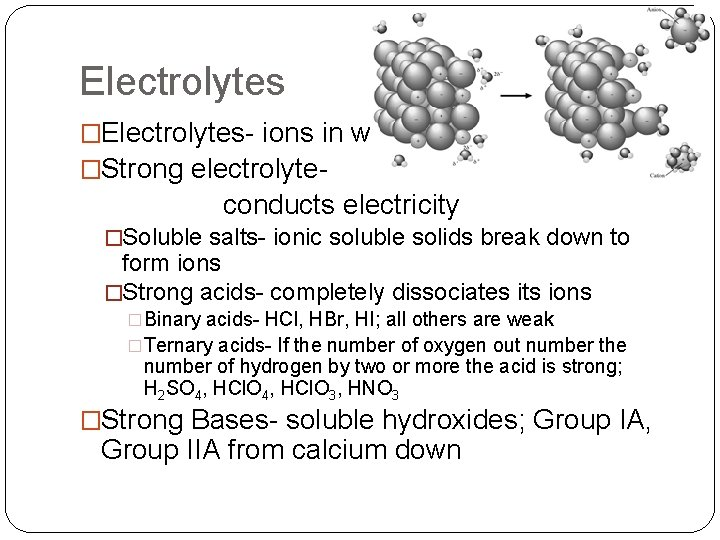Electrolytes �Electrolytes- ions in water �Strong electrolyte- conducts electricity �Soluble salts- ionic soluble solids break down to form ions �Strong acids- completely dissociates its ions �Binary acids- HCl, HBr, HI; all others are weak �Ternary acids- If the number of oxygen out number the number of hydrogen by two or more the acid is strong; H 2 SO 4, HCl. O 3, HNO 3 �Strong Bases- soluble hydroxides; Group IA, Group IIA from calcium downWeak and Non-electrolytes Weak electrolyte- weakly conducts electricity o Weak acids o Weak bases Nonelectrolyte- does not conduct electricity o Highly insoluble saltsArrhenius Definition �Arrhenius �Acid- substance containing ionizable H+ �Base- contains OH�Ex. Write an equation for the dissociation of sodium hydroxide. �Ex. Write and equation for the dissociation of acetic acid.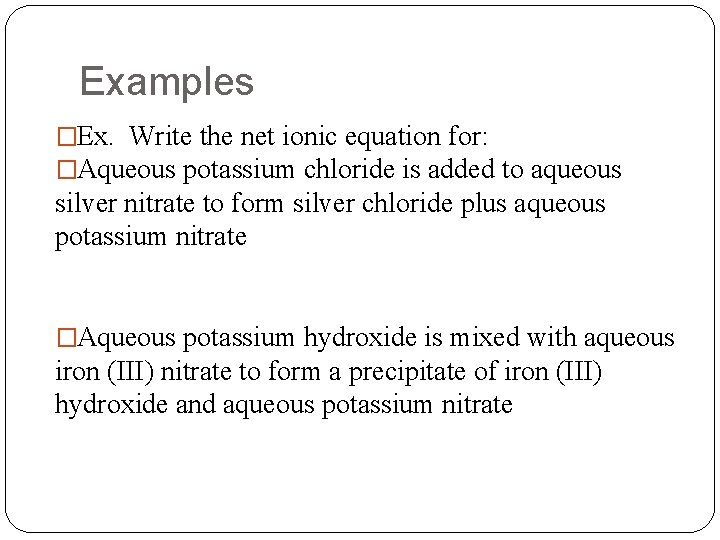Examples �Ex. Write the net ionic equation for: �Aqueous potassium chloride is added to aqueous silver nitrate to form silver chloride plus aqueous potassium nitrate �Aqueous potassium hydroxide is mixed with aqueous iron (III) nitrate to form a precipitate of iron (III) hydroxide and aqueous potassium nitrate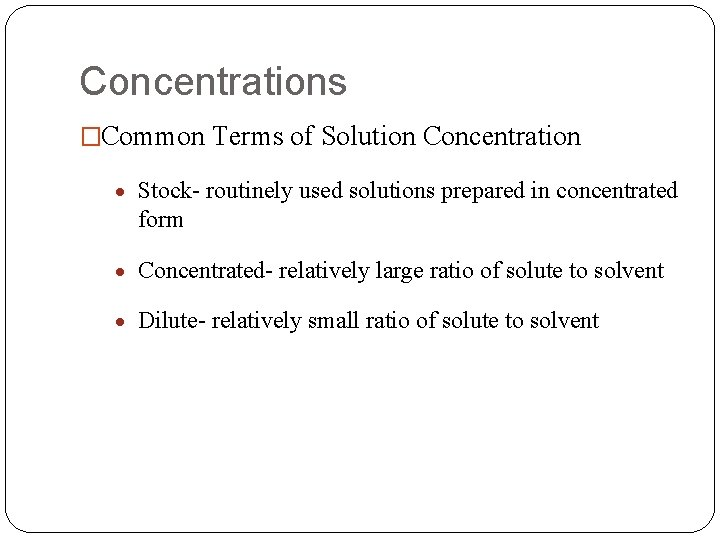Concentrations �Common Terms of Solution Concentration Stock- routinely used solutions prepared in concentrated form Concentrated- relatively large ratio of solute to solvent Dilute- relatively small ratio of solute to solvent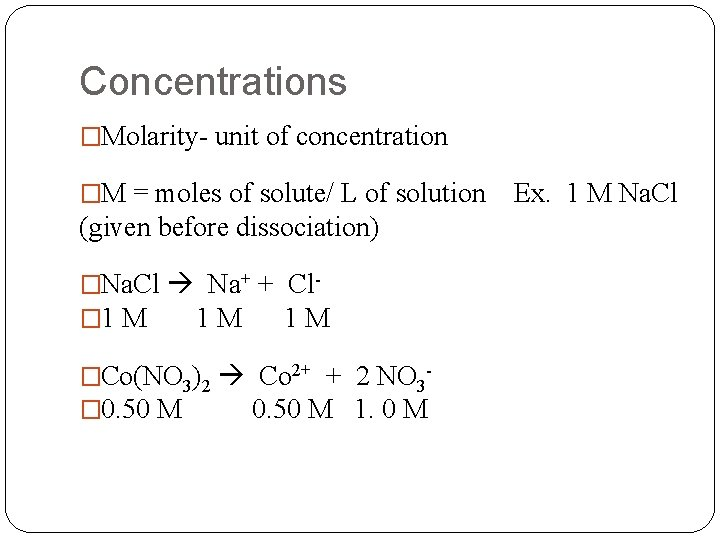Concentrations �Molarity- unit of concentration �M = moles of solute/ L of solution Ex. 1 M Na. Cl (given before dissociation) �Na. Cl Na+ + Cl� 1 M 1 M �Co(NO 3)2 Co 2+ + 2 NO 3� 0. 50 M 1. 0 MExample �Ex. What are the concentrations of each ion in a solution of 0. 32 M Ba(NO 3)2?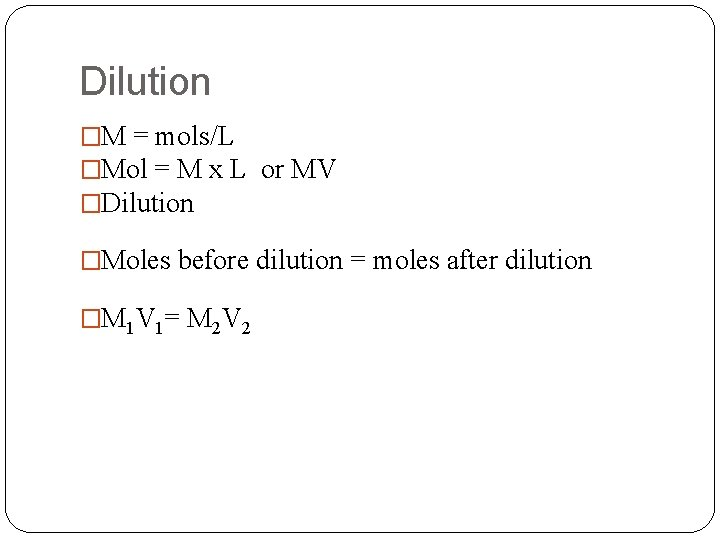Dilution �M = mols/L �Mol = M x L or MV �Dilution �Moles before dilution = moles after dilution �M 1 V 1= M 2 V 2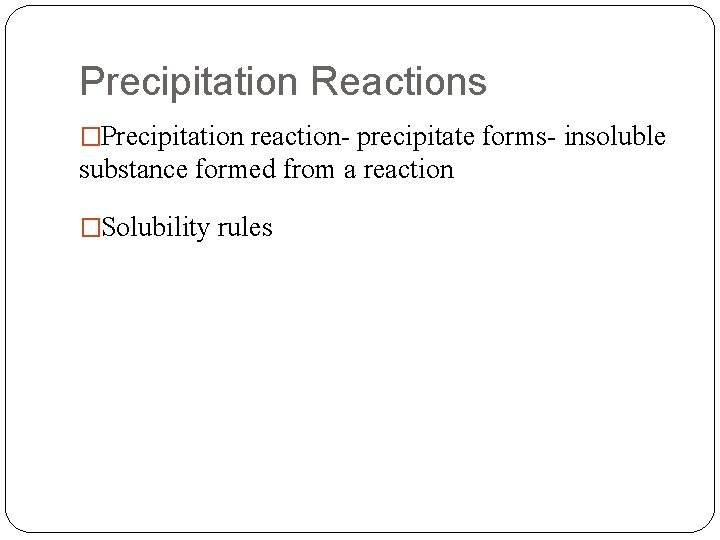Precipitation Reactions �Precipitation reaction- precipitate forms- insoluble substance formed from a reaction �Solubility rulesConcentration �Weight percent- concentration unit; used in medical field �# of grams of solute/ 100 g of water �Ex. How would you prepare a 5% glucose solution?Net Ionic Equations Molecular Equation Ionic Equation Net Ionic Equation Spectator IonsStoichiometry of Chemical Reactions �Ex. Calculate the mass of solid KCl necessary to precipitate all of the Ag+ ions from a solution of 0. 75 L of a 0. 15 M Ag. NO 3 solution.Stoichiometry �Limiting Reactant- Must take into consideration which one is limiting. �Ex. When aqueous solutions of Na 2 SO 4 and Pb(NO 3)2 are mixed, Pb. SO 4 precipitates. Calculate the mass of Pb. SO 4 formed when 1. 25 L of 0. 0500 M Pb(NO 3)2 and 2. 00 L of 0. 0250 M Na 2 SO 4 are mixed.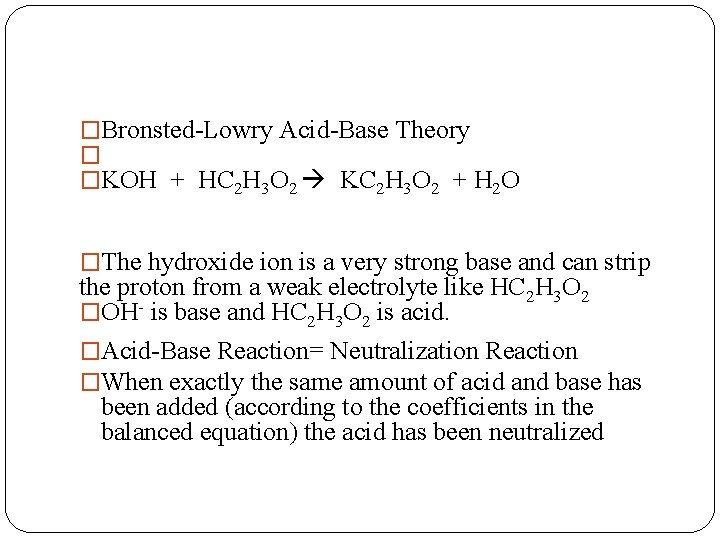�Bronsted-Lowry Acid-Base Theory � �KOH + HC 2 H 3 O 2 KC 2 H 3 O 2 + H 2 O �The hydroxide ion is a very strong base and can strip the proton from a weak electrolyte like HC 2 H 3 O 2 �OH- is base and HC 2 H 3 O 2 is acid. �Acid-Base Reaction= Neutralization Reaction �When exactly the same amount of acid and base has been added (according to the coefficients in the balanced equation) the acid has been neutralizedExample �Ex. What volume of 0. 100 M HCl solution is needed to neutralize 25. 0 m. L of a 0. 350 M Na. OH solution?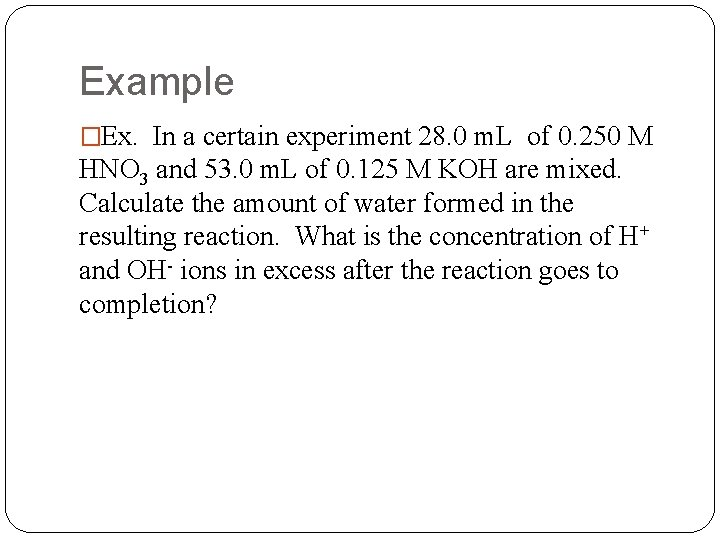Example �Ex. In a certain experiment 28. 0 m. L of 0. 250 M HNO 3 and 53. 0 m. L of 0. 125 M KOH are mixed. Calculate the amount of water formed in the resulting reaction. What is the concentration of H+ and OH- ions in excess after the reaction goes to completion?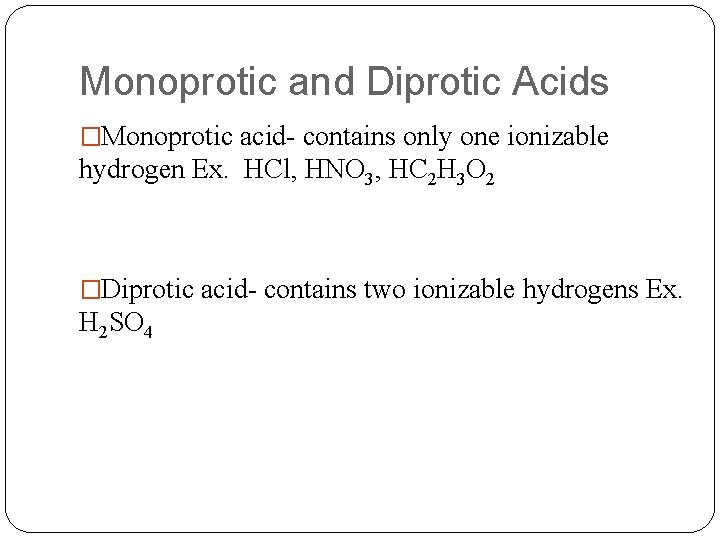Monoprotic and Diprotic Acids �Monoprotic acid- contains only one ionizable hydrogen Ex. HCl, HNO 3, HC 2 H 3 O 2 �Diprotic acid- contains two ionizable hydrogens Ex. H 2 SO 4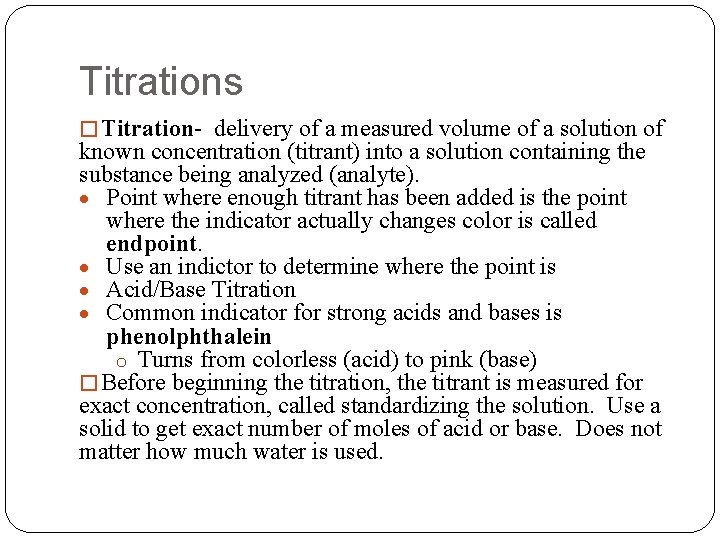Titrations � Titration- delivery of a measured volume of a solution of known concentration (titrant) into a solution containing the substance being analyzed (analyte). Point where enough titrant has been added is the point where the indicator actually changes color is called endpoint. Use an indictor to determine where the point is Acid/Base Titration Common indicator for strong acids and bases is phenolphthalein o Turns from colorless (acid) to pink (base) � Before beginning the titration, the titrant is measured for exact concentration, called standardizing the solution. Use a solid to get exact number of moles of acid or base. Does not matter how much water is used.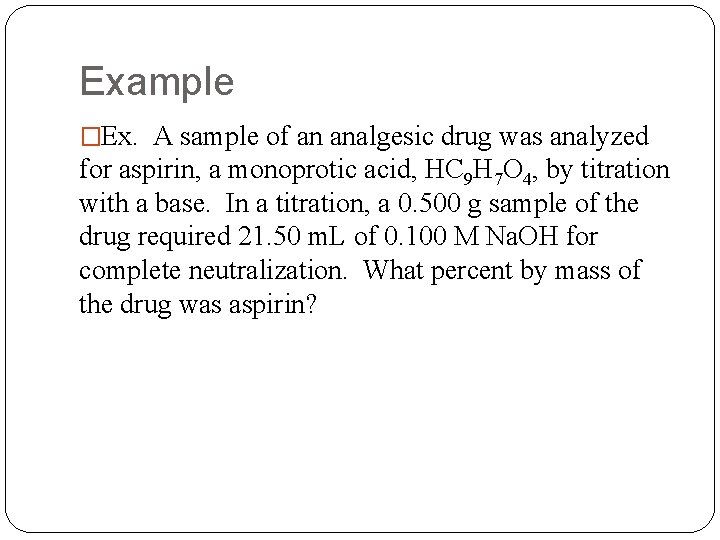Example �Ex. A sample of an analgesic drug was analyzed for aspirin, a monoprotic acid, HC 9 H 7 O 4, by titration with a base. In a titration, a 0. 500 g sample of the drug required 21. 50 m. L of 0. 100 M Na. OH for complete neutralization. What percent by mass of the drug was aspirin?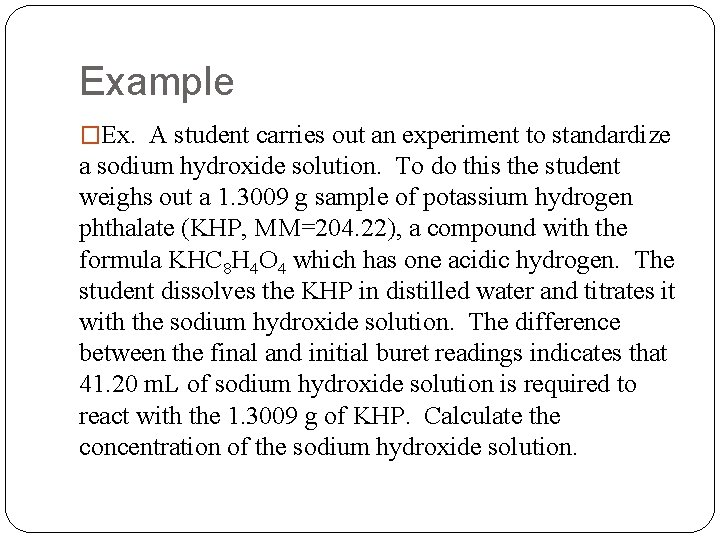Example �Ex. A student carries out an experiment to standardize a sodium hydroxide solution. To do this the student weighs out a 1. 3009 g sample of potassium hydrogen phthalate (KHP, MM=204. 22), a compound with the formula KHC 8 H 4 O 4 which has one acidic hydrogen. The student dissolves the KHP in distilled water and titrates it with the sodium hydroxide solution. The difference between the final and initial buret readings indicates that 41. 20 m. L of sodium hydroxide solution is required to react with the 1. 3009 g of KHP. Calculate the concentration of the sodium hydroxide solution.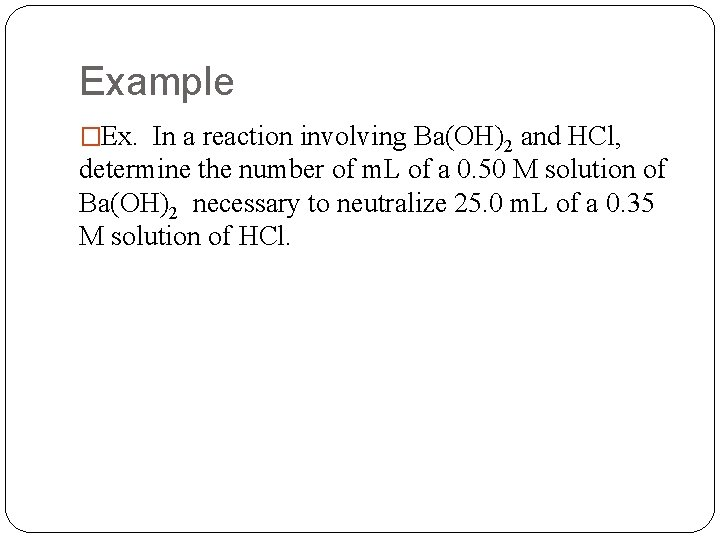Example �Ex. In a reaction involving Ba(OH)2 and HCl, determine the number of m. L of a 0. 50 M solution of Ba(OH)2 necessary to neutralize 25. 0 m. L of a 0. 35 M solution of HCl.Redox �Electrons are transferred- called oxidation- reduction reactions or redox. �Use oxidation numbers (states) to keep track of electron transfer.Oxidation numbers � Rules for assigning oxidation states: 1. The oxidation state of an atom in an element is 0. 2. The oxidation state of a monatomic ion is the same as its 3. 4. 5. 6. charge. In its compounds, fluorine is always assigned an oxidation state of – 1. Oxygen is usually assigned an oxidation state of – 2 in its covalent compounds. Exceptions are peroxides O 22 - and in OF 2 where oxygen is +2. In its covalent compounds with nonmetals, hydrogen is assigned an oxidation state of +1. The sum of the oxidation states must be 0 for an electrically neutral compound. For an ion, the sum of the charges must equal the charge on the ion.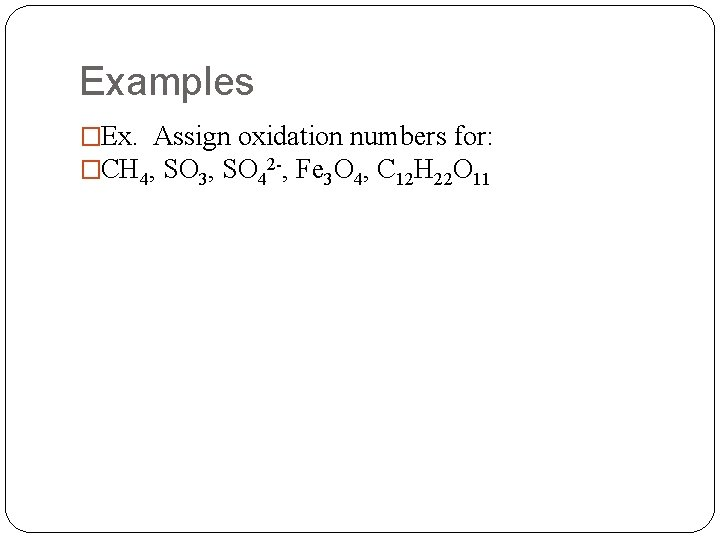Examples �Ex. Assign oxidation numbers for: �CH 4, SO 3, SO 42 -, Fe 3 O 4, C 12 H 22 O 11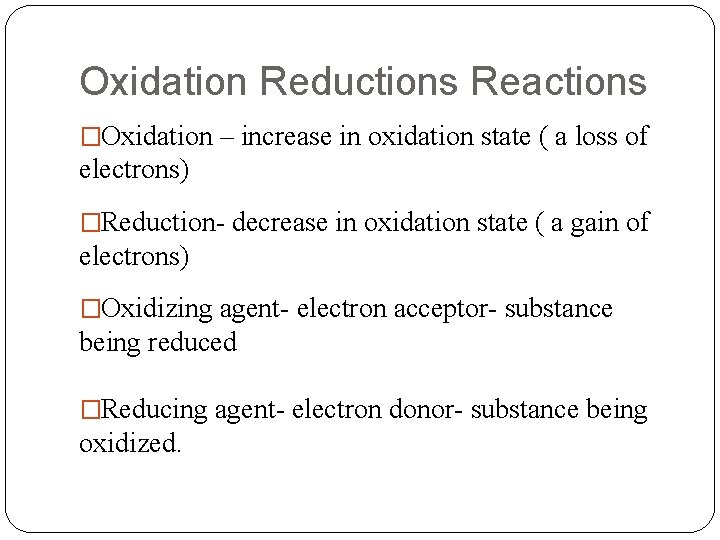Oxidation Reductions Reactions �Oxidation – increase in oxidation state ( a loss of electrons) �Reduction- decrease in oxidation state ( a gain of electrons) �Oxidizing agent- electron acceptor- substance being reduced �Reducing agent- electron donor- substance being oxidized.Redox �When identifying what is oxidized or reduced use specific element. �When identifying oxidizing or reducing agent identify whole compound.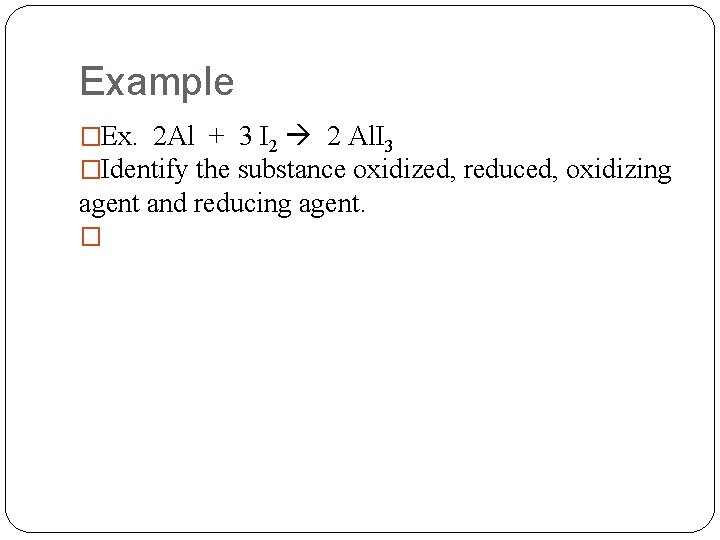Example �Ex. 2 Al + 3 I 2 2 Al. I 3 �Identify the substance oxidized, reduced, oxidizing agent and reducing agent. �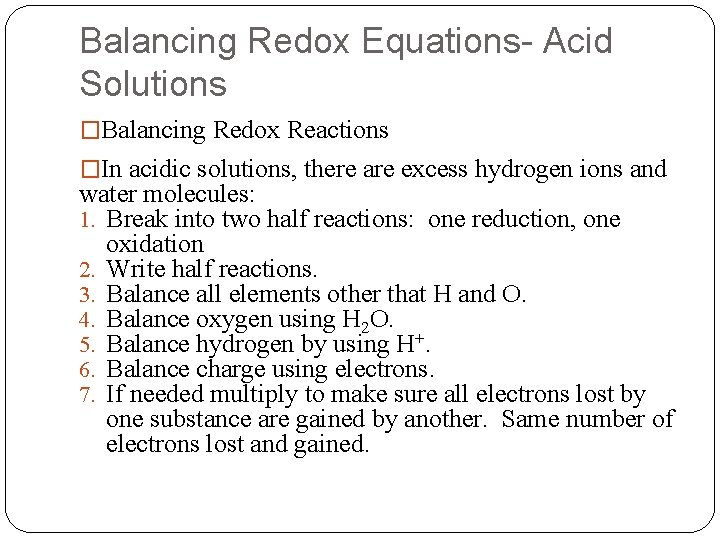Balancing Redox Equations- Acid Solutions �Balancing Redox Reactions �In acidic solutions, there are excess hydrogen ions and water molecules: 1. Break into two half reactions: one reduction, one oxidation 2. Write half reactions. 3. Balance all elements other that H and O. 4. Balance oxygen using H 2 O. 5. Balance hydrogen by using H+. 6. Balance charge using electrons. 7. If needed multiply to make sure all electrons lost by one substance are gained by another. Same number of electrons lost and gained.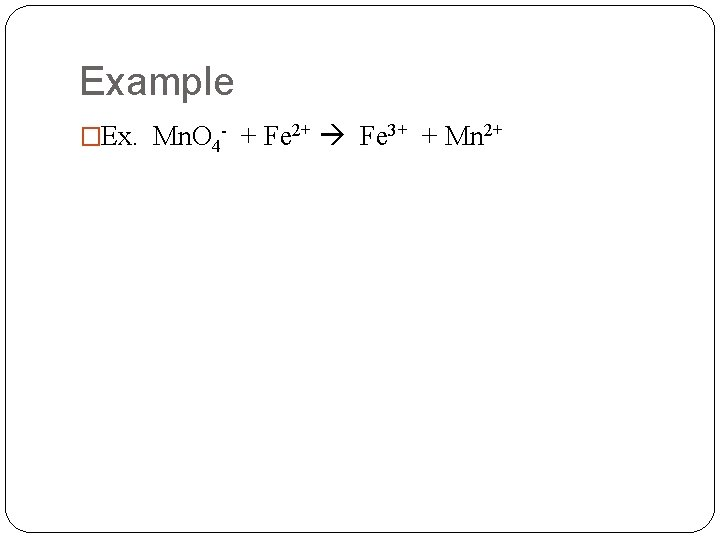Example �Ex. Mn. O 4 - + Fe 2+ Fe 3+ + Mn 2+Balancing Redox Equations- Basic Solutions �In basic solutions, there are excess hydroxide ions and water molecules: 1. Balance according to acid rules. 2. For every H+ add OH- to both sides of the equation. 3. Form water on side containing H+ and OH-. �Add together and eliminate species on both sides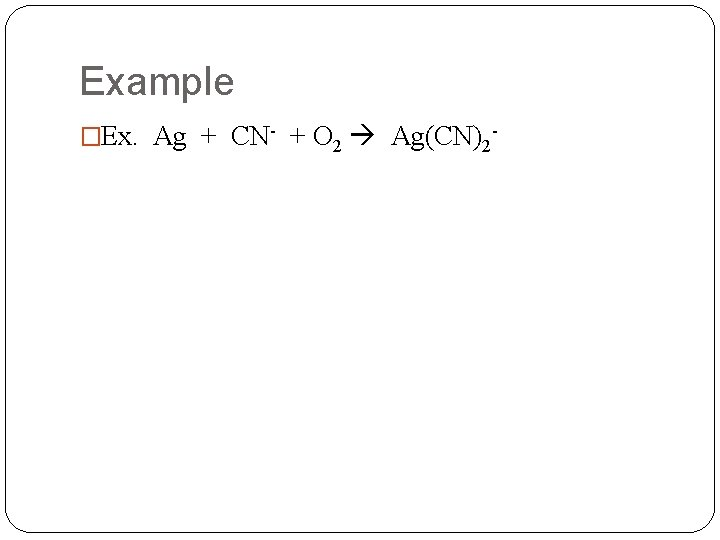Example �Ex. Ag + CN- + O 2 Ag(CN)2 -